How Cheenta works to ensure student success?
Explore the Back-Story

# ISI Entrance 2011 - B.Math Subjective Paper| Problems & SolutionsHere, you will find all the questions of ISI Entrance Paper 2011 from Indian Statistical Institute's B. Math Entrance. You will also get the solutions soon of all the previous year problems.

Problem 1 :

Let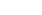be a constant such that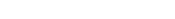for all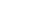What can you say about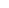? Justify your answer.

Problem 2 :

Let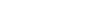for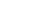. Define a function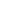on the nonnegative real numbers as follows: for each integer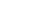, the graph of the functionon the interval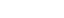is the straight line segment connecting the points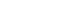and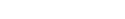. Find the total area of the region which lies between the curves of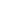and.

Problem 3 :

For any positive integer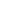, show that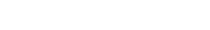.

Problem 4 :

If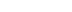are not necessarily distinct real numbers such that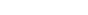for all, then show that we can choose three of them such that they are the lengths of the sides of a triangle.

Problem 5 :

For any real number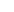let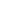denote the largest integer which is less than or equal to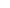. Let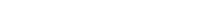be the sequence of non-square positive integers. If theth non-square positive integer satisfies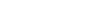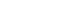then show that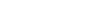Problem 6 :

Letand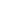be two cubes with sides of lengths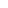andrespectively, whereandare positive integers. Show that the difference of their volumes equals the difference of their surface areas, if and only if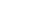.

Problem 7 :

Let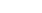be any triangle and letbe a point on the line segment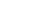Show that there exists a line parallel to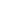which divides the triangleinto two equal parts of equal area.

Problem 8 :

Let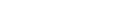be real numbers, and consider the function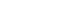be given by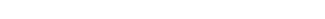Show that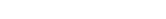Here, you will find all the questions of ISI Entrance Paper 2011 from Indian Statistical Institute's B. Math Entrance. You will also get the solutions soon of all the previous year problems.

Problem 1 :

Letbe a constant such thatfor allWhat can you say about? Justify your answer.

Problem 2 :

Letfor. Define a functionon the nonnegative real numbers as follows: for each integer, the graph of the functionon the intervalis the straight line segment connecting the pointsand. Find the total area of the region which lies between the curves ofand.

Problem 3 :

For any positive integer, show that.

Problem 4 :

Ifare not necessarily distinct real numbers such thatfor all, then show that we can choose three of them such that they are the lengths of the sides of a triangle.

Problem 5 :

For any real numberletdenote the largest integer which is less than or equal to. Letbe the sequence of non-square positive integers. If theth non-square positive integer satisfiesthen show thatProblem 6 :

Letandbe two cubes with sides of lengthsandrespectively, whereandare positive integers. Show that the difference of their volumes equals the difference of their surface areas, if and only if.

Problem 7 :

Letbe any triangle and letbe a point on the line segmentShow that there exists a line parallel towhich divides the triangleinto two equal parts of equal area.

Problem 8 :

Letbe real numbers, and consider the functionbe given byShow thatThis site uses Akismet to reduce spam. Learn how your comment data is processed.

### Knowledge Partner